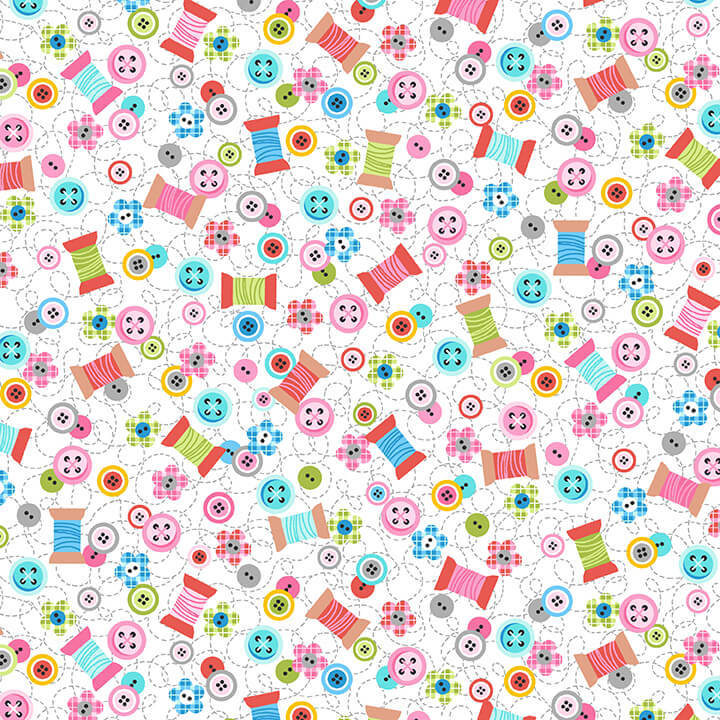# The story behind Sew Kind

At 11 years of age, Charlotte Gould is Studio E's youngest licensor ever and she has such an amazing story behind her first fabric collection.

Charlotte was born with a cleft lip and palate for which she has had several surgeries, including two before her first birthday.
Her company, Stitches by Charlotte, was created when Charlotte received her first sewing machine for Christmas.
With the sewing machine, she made a doll that looked like her with long brown hair, glasses and a sweet smile with a tiny scar.
She received so much positive feedback about her perfectly “imperfect” doll that she began to make dolls for other children who were going through scary surgeries too.
Charlotte’s Sew Kind collection for Studio E is about her love of sewing in order to make others smile.
The collection features a 36” panel with a doll form that looks like Charlotte.
5% of every sale benefits the Operation Smile Charity so smile knowing you did something kind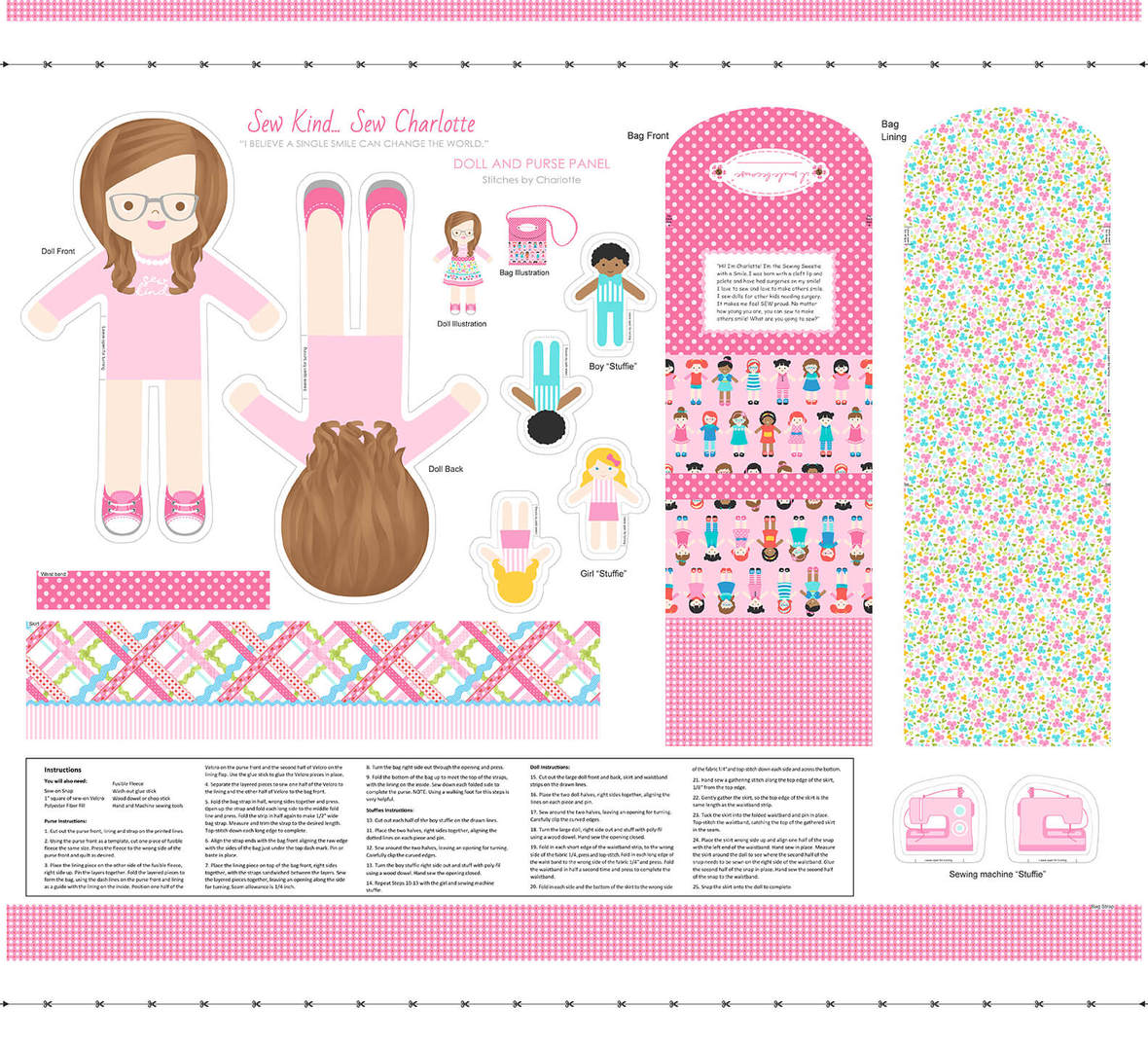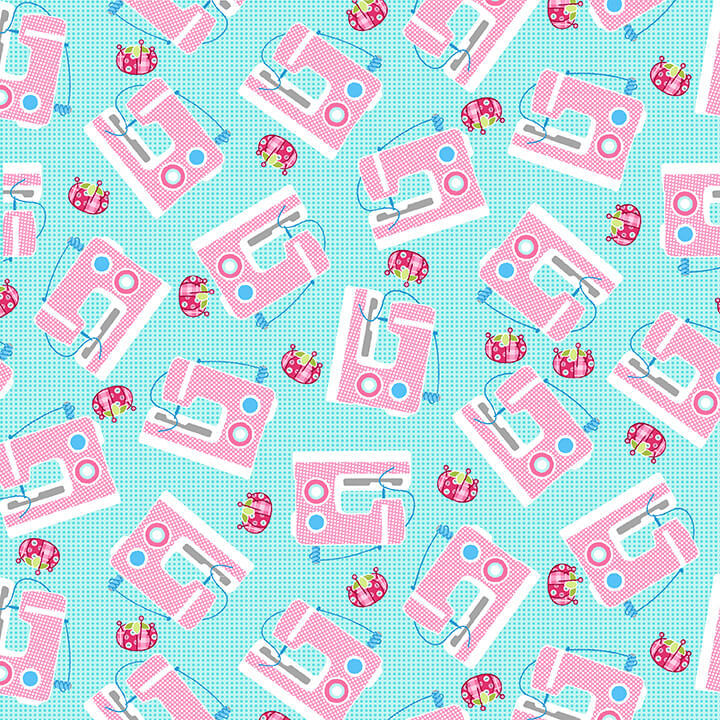table div table+table+table+table+table+table div table{width:100%;padding:0}table div table+table+table+table+table+table div table img{width:96.23%;padding:0;float:none}table div table+table+table+table+table+table div table td{width:100%;padding:0 1.88% 18px}/* styles */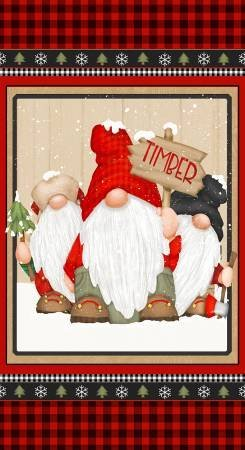# Timber Gnomes!

The gnomes are back with a wintery theme!
This line has been very popular, so don't wait too long to get yours!

 table div table+table+table+table+table+table+table+table+table div table{width:100%;padding:0}table div table+table+table+table+table+table+table+table+table div table img{width:96.23%;padding:0;float:none}table div table+table+table+table+table+table+table+table+table div table td{width:100%;padding:0 1.88% 18px}/* styles */# The View From Here

If you can't live at the beach, you can at least create your own view of the beach!
This fabric is digitally printed with amazing detail, check out the water and sand.

 table div table+table+table+table+table+table+table+table+table+table+table+table div table{width:100%;padding:0}table div table+table+table+table+table+table+table+table+table+table+table+table div table img{width:96.23%;padding:0;float:none}table div table+table+table+table+table+table+table+table+table+table+table+table div table td{width:100%;padding:0 1.88% 18px}/* styles */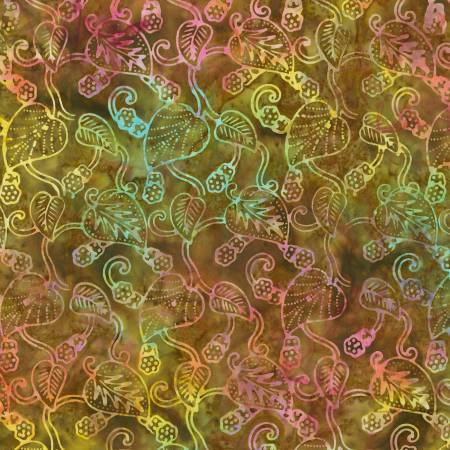# New Batiks!

It's always time for new Batiks, don't you think?
Lots of great greens in this batch and an interesting grid pattern.
Be sure to check them all out!

 table div table+table+table+table+table+table+table+table+table+table+table+table+table+table+table div table{width:100%;padding:0}table div table+table+table+table+table+table+table+table+table+table+table+table+table+table+table div table img{width:96.23%;padding:0;float:none}table div table+table+table+table+table+table+table+table+table+table+table+table+table+table+table div table td{width:100%;padding:0 1.88% 18px}/* styles */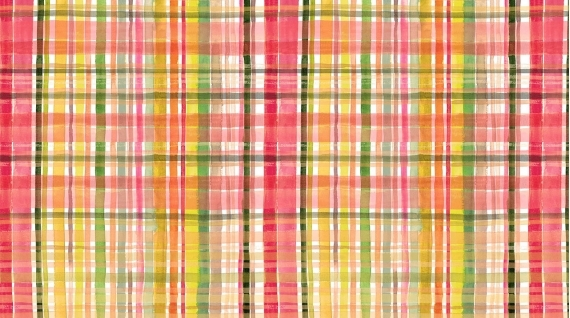New Fabrics!
Lots of great blenders arrived, perfect for your fall and holiday projects, lots of red and green.
This awesome plaid and be sure to take a look at the periodic table fabric - I bet you know a science lover who would love you to make something with that fabric!

 table div table+table+table+table+table+table+table+table+table+table+table+table+table+table+table+table+table+table div table{width:100%;padding:0}table div table+table+table+table+table+table+table+table+table+table+table+table+table+table+table+table+table+table div table img{width:96.23%;padding:0;float:none}table div table+table+table+table+table+table+table+table+table+table+table+table+table+table+table+table+table+table div table td{width:100%;padding:0 1.88% 18px}/* styles */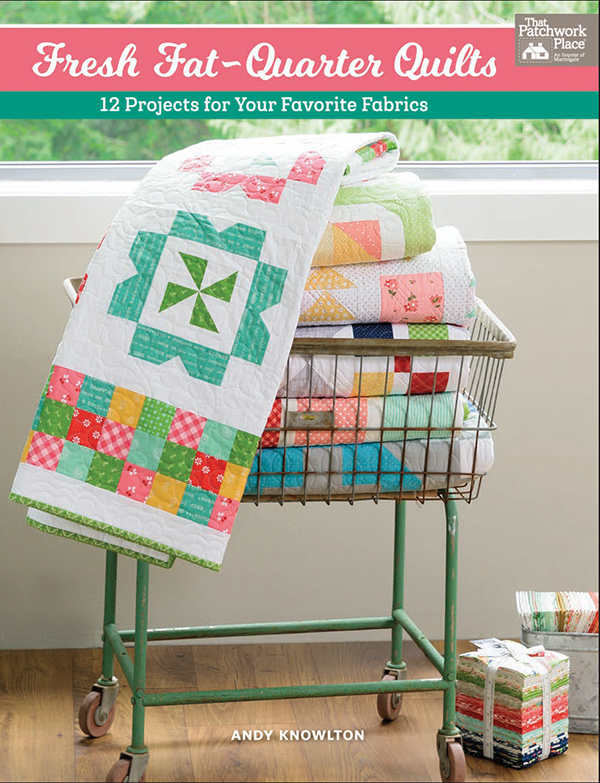# New Goodies!

Great fat quarter book with projects using 6, 8, 10, 12 or 14 fat quarters!
New Elizabeth Hartman patterns!
Creative Grids Kitty Cornered Ruler makes 11 sizes of the kite block and there is a fall runner pattern to try it out with!
Cute change purse (or for m&m's, I won't judge)
AND
Did you know it is the 20th anniversary of Atkinson Designs Yellow Brick Road pattern?
Every quilter needs to make a least one Yellow Brick Road quilt, and it probably won't be your last!
Terry designed Mini Brick Road which is sure to be a winner! Uses charm packs (and I know you have a couple of those around) or cut your own. This pattern promises to be another go-to pattern!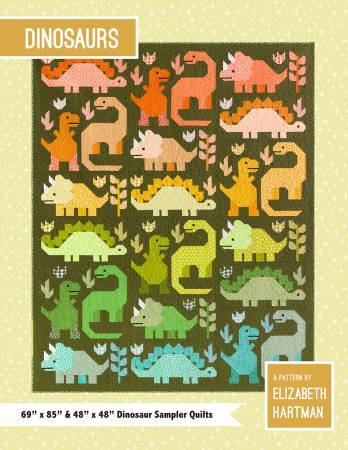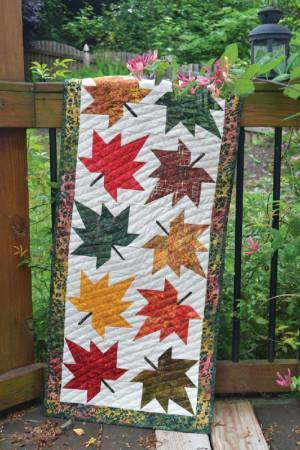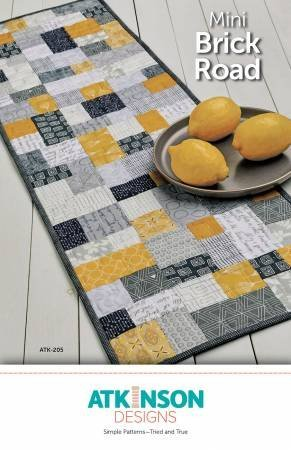table div table+table+table+table+table+table+table+table+table+table+table+table+table+table+table+table+table+table+table+table+table+table div table{width:100%;padding:0}table div table+table+table+table+table+table+table+table+table+table+table+table+table+table+table+table+table+table+table+table+table+table div table img{width:96.23%;padding:0;float:none}table div table+table+table+table+table+table+table+table+table+table+table+table+table+table+table+table+table+table+table+table+table+table div table td{width:100%;padding:0 1.88% 18px}/* styles */# Quilter's Trek!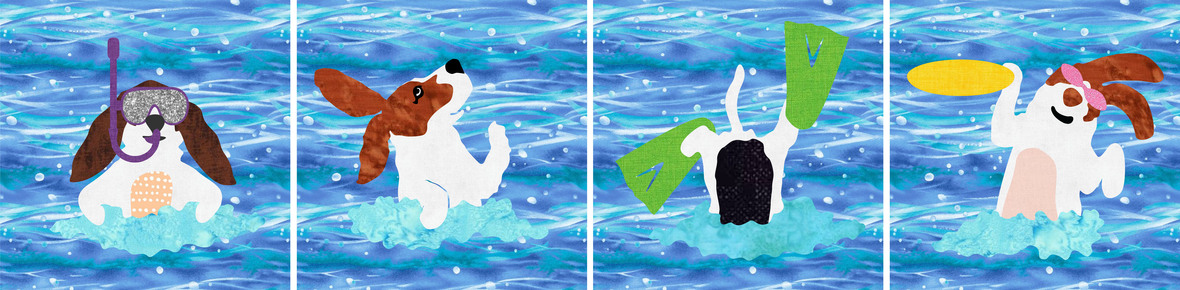/* styles */ It's the Dippin' Dawgs! The theme this year is the color blue Shops were to design 4 blocks that finish at 9" square Blocks can be put together as a row, a square, or used individually. So Freddie, Maggie, Stanley and Lulu are having a day at the beach!
 table div table+table+table+table+table+table+table+table+table+table+table+table+table+table+table+table+table+table+table+table+table+table+table+table+table+table+table div table{width:100%;padding:0}table div table+table+table+table+table+table+table+table+table+table+table+table+table+table+table+table+table+table+table+table+table+table+table+table+table+table+table div table img{width:96.23%;padding:0;float:none}table div table+table+table+table+table+table+table+table+table+table+table+table+table+table+table+table+table+table+table+table+table+table+table+table+table+table+table div table td{width:100%;padding:0 1.88% 18px}/* styles */## We have just 2 kits left for Machine Embroidery!

Psssst ... have you heard? Kimberbell's Love Notes Mystery Quilt is happening at Cotton Patch this summer!
Available for both sewing and machine embroidery, the Love Notes Mystery Quilt is an exciting way to experience the joy of creativity™ with other stitching enthusiasts starting August 3rd.
Kits include Kimberbell fabrics and Kimberbell embellishments to add that special touch in a super cute custom Kimberbell box, plus your clues are sent to you for 5 weeks in love notes!
Kits are limited, so reserve yours today, and each participant will be sent a free project in June to stitch while we wait for the mystery to start.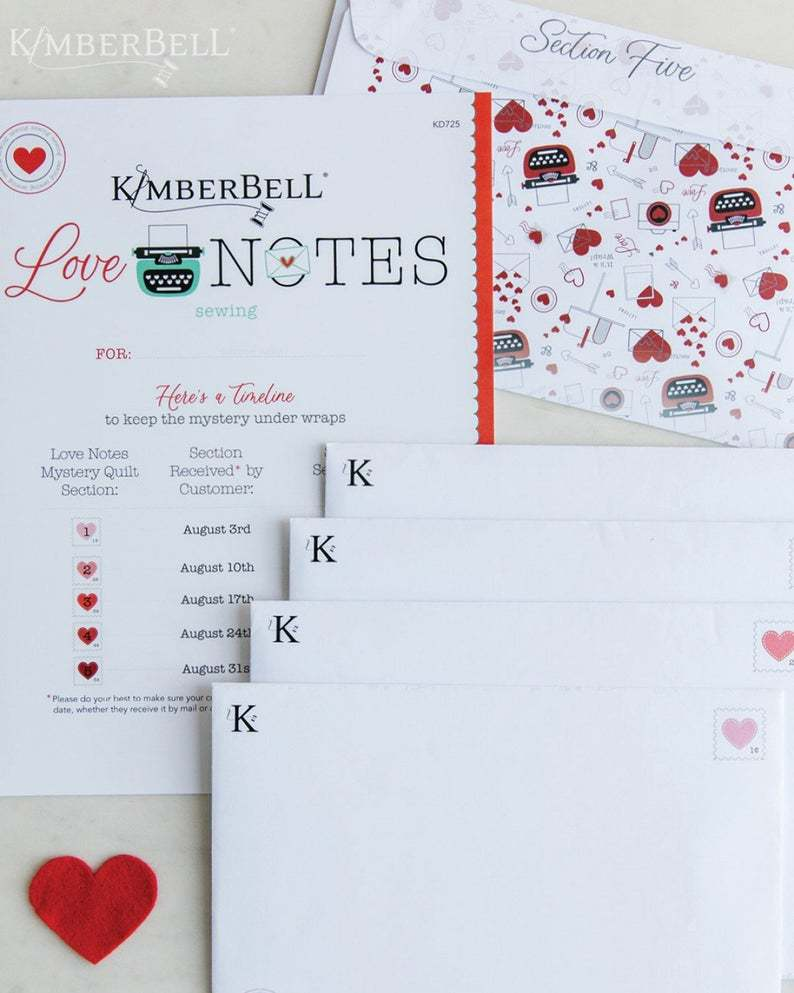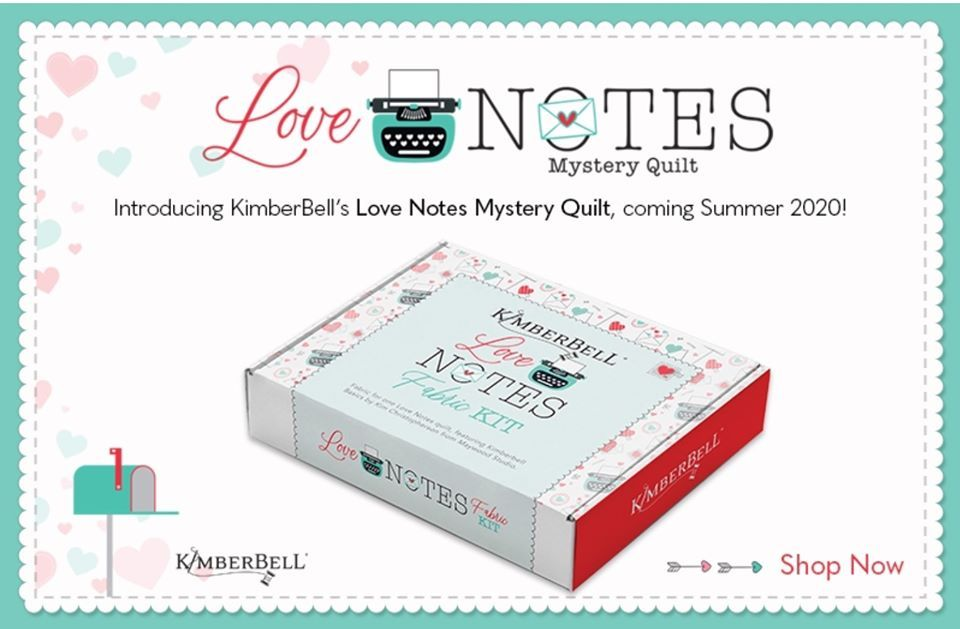table div table+table+table+table+table+table+table+table+table+table+table+table+table+table+table+table+table+table+table+table+table+table+table+table+table+table+table+table+table+table+table+table div table{width:100%;padding:0}table div table+table+table+table+table+table+table+table+table+table+table+table+table+table+table+table+table+table+table+table+table+table+table+table+table+table+table+table+table+table+table+table div table img{width:96.23%;padding:0;float:none}table div table+table+table+table+table+table+table+table+table+table+table+table+table+table+table+table+table+table+table+table+table+table+table+table+table+table+table+table+table+table+table+table div table td{width:100%;padding:0 1.88% 18px}/* styles */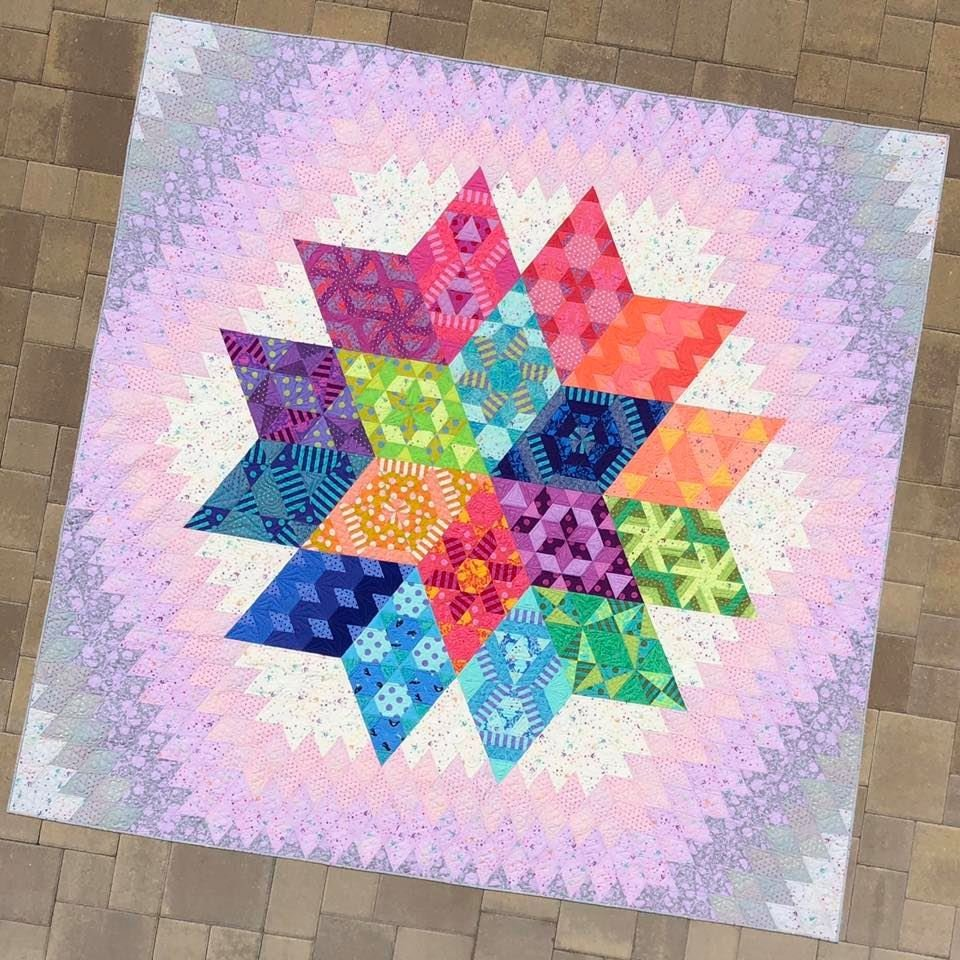# Nebula Block of the Month

Reserve your spot in this 10 month program beginning in January 2021!

Create 9 unique blocks using, are you ready for this, 74 different Tula Pink fabrics and 7 Tula Pink solids!

Pattern is by Jaybird Quilts and there are NO Y-Seams!

## Remember to sign up before July 31 and receive an exclusive Tula Pink project bag with a Nebula Label!

 table div table+table+table+table+table+table+table+table+table+table+table+table+table+table+table+table+table+table+table+table+table+table+table+table+table+table+table+table+table+table+table+table+table+table+table div table{width:100%;padding:0}table div table+table+table+table+table+table+table+table+table+table+table+table+table+table+table+table+table+table+table+table+table+table+table+table+table+table+table+table+table+table+table+table+table+table+table div table img{width:96.23%;padding:0;float:none}table div table+table+table+table+table+table+table+table+table+table+table+table+table+table+table+table+table+table+table+table+table+table+table+table+table+table+table+table+table+table+table+table+table+table+table div table td{width:100%;padding:0 1.88% 18px}/* styles */# FB Live is the place to be Sunday at 7pm!

You will be the 1st to see new fabrics, notions, kits and other great things that you need to know about!

## We put together fun kits that often sell out that very night - you don't want to miss out!

There will be prizes, live demos and special pricing!

You will be able to purchase during the Live Broadcast and we can ship - sort of like Stuff Your Stocking but all in one night.
If you miss the FB Live - not to worry!

## You can view the replay on our FB Page AND you can still purchase at the special prices until 3pm Monday!

We will go "live" at 6:55 and see what the hounds are up to, then
start the Soiree at 7pm (Eastern)

## Join me for a fun evening!

 table div table+table+table+table+table+table+table+table+table+table+table+table+table+table+table+table+table+table+table+table+table+table+table+table+table+table+table+table+table+table+table+table+table+table+table+table+table+table+table+table div table{width:100%;padding:0}table div table+table+table+table+table+table+table+table+table+table+table+table+table+table+table+table+table+table+table+table+table+table+table+table+table+table+table+table+table+table+table+table+table+table+table+table+table+table+table+table div table img{width:96.23%;padding:0;float:none}table div table+table+table+table+table+table+table+table+table+table+table+table+table+table+table+table+table+table+table+table+table+table+table+table+table+table+table+table+table+table+table+table+table+table+table+table+table+table+table+table div table td{width:100%;padding:0 1.88% 18px}/* styles */# Meet me in the Club House!

Cotton Patch Club House is a new FaceBook group and we want you to be a part of it!
You will find:
An online community for friends of Cotton Patch Quilt Shop!
A place to chat and share photos of your projects.
A place to ask quilty questions, share tips and demos
A place for you to find sneak peeks and early access to items on our FaceBook Live Sunday Soiree!

 table div table+table+table+table+table+table+table+table+table+table+table+table+table+table+table+table+table+table+table+table+table+table+table+table+table+table+table+table+table+table+table+table+table+table+table+table+table+table+table+table+table+table+table div table{width:100%;padding:0}table div table+table+table+table+table+table+table+table+table+table+table+table+table+table+table+table+table+table+table+table+table+table+table+table+table+table+table+table+table+table+table+table+table+table+table+table+table+table+table+table+table+table+table div table img{width:96.23%;padding:0;float:none}table div table+table+table+table+table+table+table+table+table+table+table+table+table+table+table+table+table+table+table+table+table+table+table+table+table+table+table+table+table+table+table+table+table+table+table+table+table+table+table+table+table+table+table div table td{width:100%;padding:0 1.88% 18px}/* styles *//* styles */ Although the Stay at Home Order has been lifted in Florida, the storefront at Cotton Patch will remained closed to shopping for now. An opening date is still to be determined. Our website is open 24/7 and we are filling orders for curbside pickup or shipping right to your door! To best serve you, all orders should be placed through the website and not over the phone. Payment on the website is more secure than reading your credit card numbers over the phone and less chance for errors on items/quantity. Curbside pickup is available 10am - 2pm Tuesday through Saturday. You will get an email telling you when you order is ready for pickup. Call us from the parking lot and we will bring your purchases out to you. Thank you for your continued support with online orders and curbside pickup - we appreciate your business! We miss your smiling faces and look forward to welcoming you back soon! Laura
 table div table+table+table+table+table+table+table+table+table+table+table+table+table+table+table+table+table+table+table+table+table+table+table+table+table+table+table+table+table+table+table+table+table+table+table+table+table+table+table+table+table+table+table+table+table div table{width:100%;padding:0}table div table+table+table+table+table+table+table+table+table+table+table+table+table+table+table+table+table+table+table+table+table+table+table+table+table+table+table+table+table+table+table+table+table+table+table+table+table+table+table+table+table+table+table+table+table div table img{width:96.23%;padding:0;float:none}table div table+table+table+table+table+table+table+table+table+table+table+table+table+table+table+table+table+table+table+table+table+table+table+table+table+table+table+table+table+table+table+table+table+table+table+table+table+table+table+table+table+table+table+table+table div table td{width:100%;padding:0 1.88% 18px}/* styles *//* styles */ Stanley wants to tell you THANK YOU for your online and Sunday Soiree orders -it's keeping him in treats!
 table.module-46{width:62.83%;padding:0}table div table+table+table+table+table+table+table+table+table+table+table+table+table+table+table+table+table+table+table+table+table+table+table+table+table+table+table+table+table+table+table+table+table+table+table+table+table+table+table+table+table+table+table+table+table+table+table div table{width:62.83%;float:none;margin-left:auto;margin-right:auto;padding:0}table div table+table+table+table+table+table+table+table+table+table+table+table+table+table+table+table+table+table+table+table+table+table+table+table+table+table+table+table+table+table+table+table+table+table+table+table+table+table+table+table+table+table+table+table+table+table+table div table a{border:0 none;text-decoration:none}table div table+table+table+table+table+table+table+table+table+table+table+table+table+table+table+table+table+table+table+table+table+table+table+table+table+table+table+table+table+table+table+table+table+table+table+table+table+table+table+table+table+table+table+table+table+table+table div table img{width:100%!important;border:0 none;text-decoration:none}table div table+table+table+table+table+table+table+table+table+table+table+table+table+table+table+table+table+table+table+table+table+table+table+table+table+table+table+table+table+table+table+table+table+table+table+table+table+table+table+table+table+table+table+table+table+table+table div table td{width:100%;padding:0}/* styles */
 Like   Pin   +1